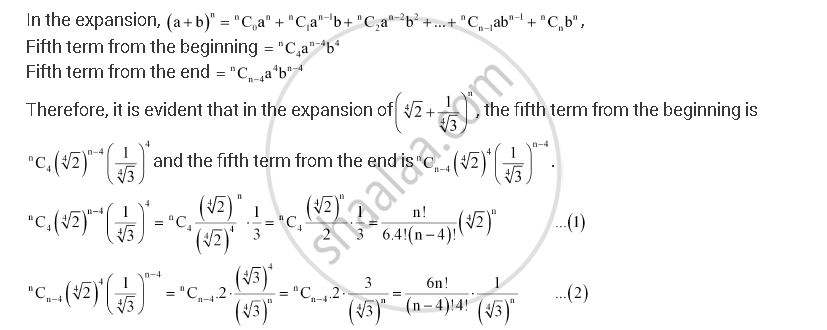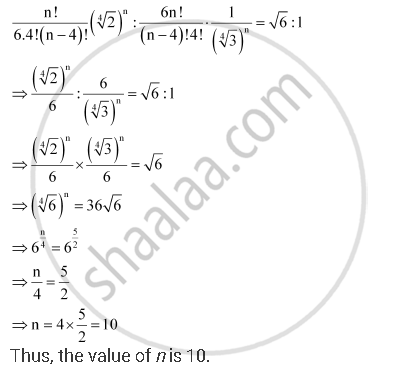Share

# Find N, If the Ratio of the Fifth Term from the Beginning to the Fifth Term from the End in the Expansion of (Root4 2 + 1/ Root4 3)^N " is " Sqrt6 : 1 - Mathematics

#### Question

Find n, if the ratio of the fifth term from the beginning to the fifth term from the end in the expansion of (root4 2 + 1/ root4 3)^n " is " sqrt6 : 1

#### SolutionIt is given that the ratio of the fifth term from the beginning to the fifth term from the end is sqrt6 : 1. Therefore, from (1) and (2), we obtainIs there an error in this question or solution?